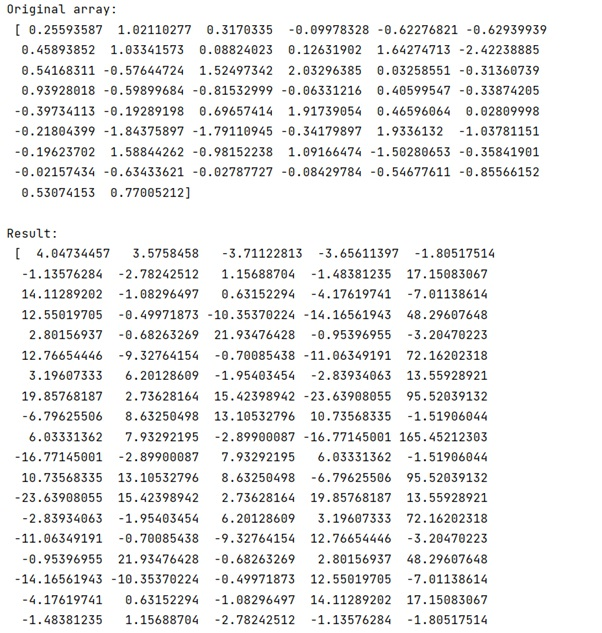# How to interpret the values returned by numpy.correlate()?

Learn, how to interpret the values returned by numpy.correlate() in Python?
Submitted by Pranit Sharma, on March 06, 2023

## Interpret the values returned by numpy.correlate()

Suppose that we are given two 1D arrays and we want to see their inter-relationships.

To interpret the values returned by numpy.correlate(), there are different procedures we can use in numpy. If we use numpy.corrcoef(arrayA, arrayB), it will give some numerical results which we need to understand. The numpy.coorelate() simply returns the cross-correlation of two vectors which can be understood with the help of an example,

## Python code to interpret the values returned by numpy.correlate()

```# Import numpy
import numpy as np

# Creating a array
arr = np.random.normal(0,1,size=50)

# Display Original array
print("Original array:\n",arr,"\n")

# Inserting a signal
arr[::10]+=5

# cross correlation
res = np.correlate(arr,arr,mode='full')

# Display result
print("Result:\n",res)
```

This will return a comb/shah function with a maximum when both data sets are overlapping. As this is an autocorrelation there will be no "lag" between the two input signals. The maximum of the correlation is therefore vector.size-1.

If we only want the value of the correlation for overlapping data, we can use mode='valid'.

Output:Languages: » C » C++ » C++ STL » Java » Data Structure » C#.Net » Android » Kotlin » SQL
Web Technologies: » PHP » Python » JavaScript » CSS » Ajax » Node.js » Web programming/HTML
Solved programs: » C » C++ » DS » Java » C#
Aptitude que. & ans.: » C » C++ » Java » DBMS
Interview que. & ans.: » C » Embedded C » Java » SEO » HR
CS Subjects: » CS Basics » O.S. » Networks » DBMS » Embedded Systems » Cloud Computing
» Machine learning » CS Organizations » Linux » DOS
More: » Articles » Puzzles » News/Updates